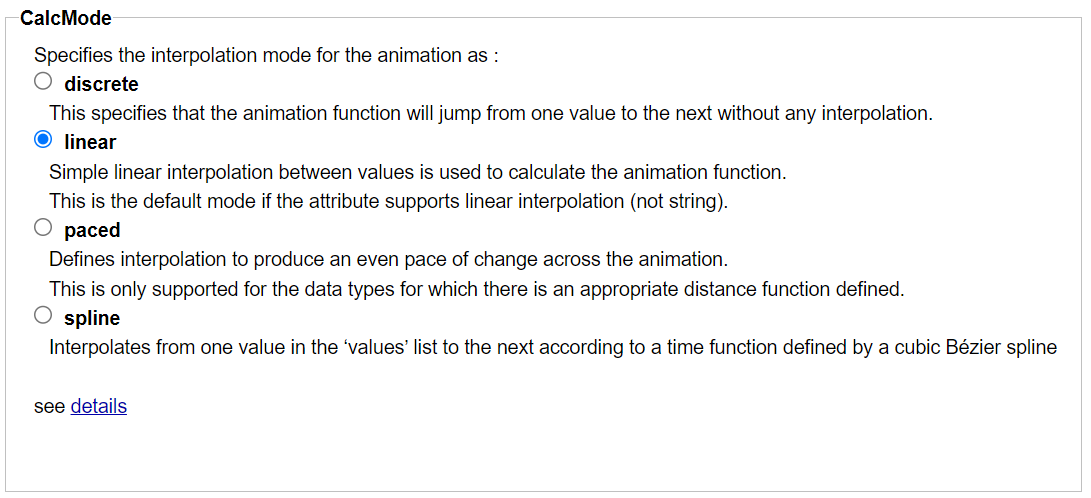## 9.5. Animation interpolation properties

They are common to animate ( attribute, transform, motion) elements and control the method to compute the value along the simple duration of the animation.

Each property has a dedicated panel that explains its behavior with links to standard specifications, and presents a simple and intuitive user interface for entering the property's value ( calcMode, keyTimes, keySplines ).

### The animate calMode property

The calcMode property define the interpolation function between :See specifications.

### The animate keyTimes property

The keyTimes property divides the simple duration into intervals to compute the variations over each :

• The value variations of the animated attribute of a animate element.

The attribute value must be defined for each interval at its origin and extremity (lists of values and keyTimes are the same size).

• The transformation matrix variations of a animate transform element.

The transformation matrix must be defined for each interval at its origin and extremity (lists of transformation values and keyTimes are the same size).

• The target element position variations along the motion path of a animate motion element.

The position along the motion path must be defined for each interval at its origin and extremity with the keyPoints property (lists of keyPoints and keyTimes are the same size).

The keyTimes property can be used to control the transition speed between values.

Each keyTimes value is a percent of the duration. The first is zero and the last is 100. The input is done with an integer value between 0 and 100 to be more convenient, finally the value is converted as a real number between 0 and 1.See animate using keyTimes showcase for an interactive demonstration.

See specifications.

### The animate keySplines property

With calMode set as spline, the keySplines property define the cubic bezier curve of the interpolation between two values that controls interval pacing.

If the animation has (n) values, keySplines has (n-1) curves. Each curve is defined with the coordinates of the 2 tangents origin and extremity (x1,y1,x2,y2).

Each coordinate is input as a positive integer value between 0 and 100 to be more convenient, then the value is converted as a real number between 0 and 1 by the task.The task draw the cubic curve with an interactive SVG document.

Each tangent point can be moved, and the curve can be selected from one drawn sample.

See animate using keySplines showcase for an interactive demonstration.

See specifications.